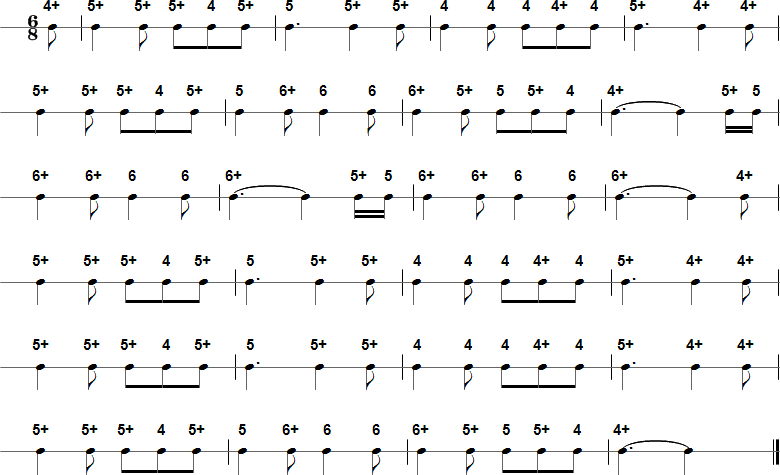Browse by Title:

# For He's a Jolly Good Fellow - Harmonica Tablature and Sheet Music## Details

• Title(s): For He's a Jolly Good Fellow

## Tab in Text Format

4+ 5+ 5+ 5+ 4 5+ 5 5+ 5+ 4 4 4 4+ 4 5+ 4+ 4+ 5+ 5+ 5+ 4 5+ 5 6+ 6 6 6+ 5+ 5 5+ 4 4+ 5+ 5 6+ 6+ 6 6 6+ 5+ 5 6+ 6+ 6 6 6+ 4+ 5+ 5+ 5+ 4 5+ 5 5+ 5+ 4 4 4 4+ 4 5+ 4+ 4+ 5+ 5+ 5+ 4 5+ 5 5+ 5+ 4 4 4 4+ 4 5+ 4+ 4+ 5+ 5+ 5+ 4 5+ 5 6+ 6 6 6+ 5+ 5 5+ 4 4+